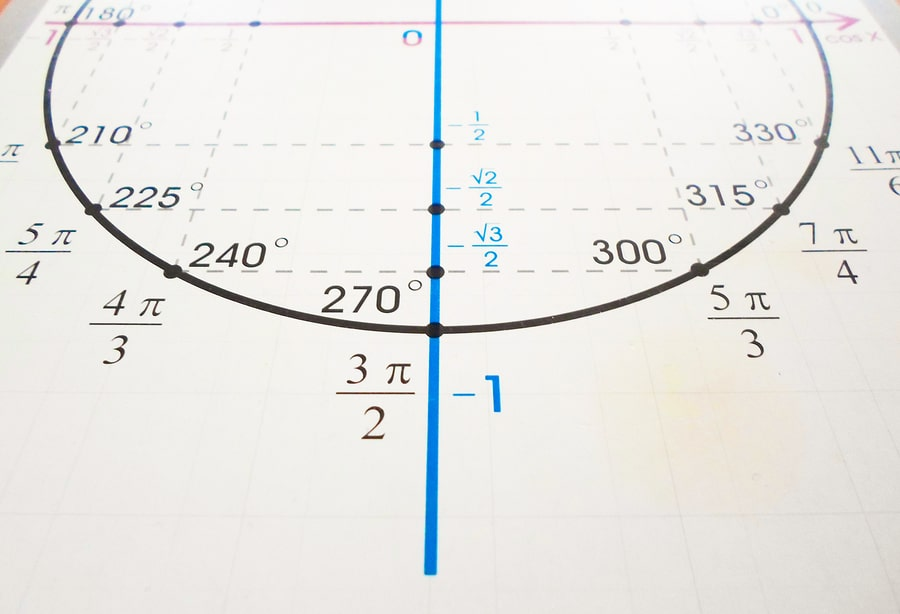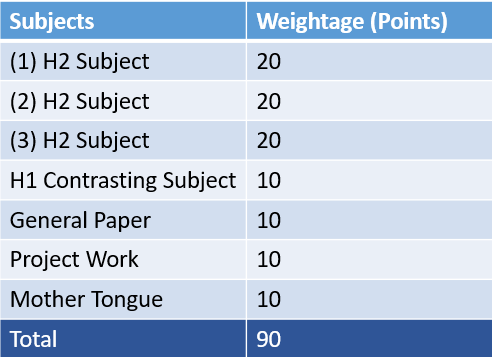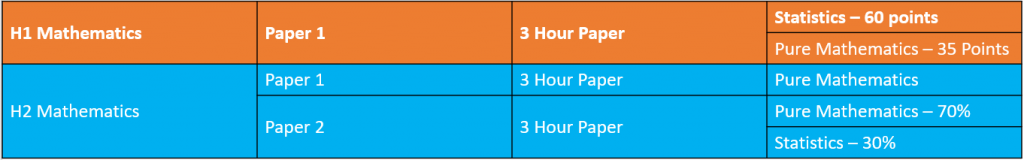# What is H2 Mathematics?## 18 Nov What is H2 Mathematics?

H2 Mathematics which stands for “Higher-2 Mathematics”, is a subject offered by junior colleges (JC) in Singapore. H2 math is an important subject for students with aspirations to take university courses such as medicine, dentistry, engineering, architecture and economics. Topics covered include functions & graphs, sequences & series, vectors, complex numbers, calculus, probability and statistics.

• What is H1, H2, H3 subjects in JC
• The importance of H2 Mathematics
• The main differences between H1 & H2 mathematics
• The jump from secondary school maths to H2 maths
• The subject’s usefulness in university application
• The challenges posed by H2 Mathematics

## The Differences Between H1, H2, & H3

When entering JC, students have a choice of taking a subject at H1, H2 or H3 level. These are indicative of the weight of the syllabus covered, with H1 being the lightest and H3 being the heaviest.

The required subject combination for “A” level students in Singapore is:

• 3 H2 content-based subjects
• 1 H1 content-based subject (has to be contrasting in comparison to the 3 H2 subjects)
• Compulsory H1 subjects – General Paper, Project Work, Mother Tongue

H2 subjects have a heavier weightage of 20 points as compared to H1 subjects which are worth 10 points. When added together, the maximum attainable points for JC is 90.

As you might have probably noticed, H3 subjects are not mandatory in JC, and are used to distinguish the top students in a cohort. They are taken by students to get ahead of the bell curve and thus attain an advantage in university admissions.## The Importance of H2 Mathematics

Mathematics is a fundamental subject at all levels of studies, contributing to the development of other disciplines. It is used by a variety of industries such as science, engineering, business and psychology. This is because a strong knowledge of mathematics empowers you to model, understand and solve real life problems.

According to the Ministry of Education, H2 Mathematics develops logical thinking and reasoning skills that are essential for the further learning of mathematics and its related disciplines.

The syllabus aims to enable students to:

• Acquire mathematical concepts and skills for future tertiary studies
• Be proficient in mathematical thinking, reasoning, communication and modelling skills
• Connect ideas within mathematics and apply mathematics concepts to related disciplines
• Experience and appreciate the nature of mathematics and its value in life

## The Main Differences between H1 & H2 Mathematics

The difference between H1 & H2 mathematics lies in the syllabus covered. In H1 mathematics, you would learn to understand and apply basic statistical techniques. This includes choosing suitable data, analysing data and making decisions based on the result. On the other hand, H2 mathematics trains students to perform these skill sets in more complex problems.

In more tangible terms, H1 mathematics contains 50% of the content in H2 mathematics. For example, topics on vectors, complex numbers and differential equations are not taught in H1 mathematics. Subsequently, H2 mathematics has 1 more examination paper than H1 mathematics.

However, you should be aware that this not make the individual questions in H1 mathematics easier than their H2 counterpart. Contrary to common hearsay, the questions in H1 mathematics are equally complicated. However, more topics are tested under H2 mathematics.## The Jump from Secondary School Maths to H2 Maths

A common comparison that is made between H2 and H1 maths, is that of A maths and E maths at secondary school level. How then is the jump like between taking A maths in secondary school to learning H2 maths in JC?

Other than having more advance versions of mathematical topics, the complexity is amped up by several levels when you transition from A maths to H2 maths. In addition, as H2 maths’ syllabus is designed to prepare students intended to pursue further studies in fields of engineering and science, you will be exposed to their applications and contexts.

Examples of applied contexts include kinematics, optimisation, electrical circuits, population growth, financial mathematics, standardised testing, market research and clinical research.

## H2 Mathematics’ Usefulness in University Applications

H2 mathematics is highly useful for training students to handle computations that involve heavy data and statistical analysis. As such, it would benefit you if you are aiming to take university courses in medicine, law, dentistry and economics.

On the other hand, H1 mathematics prepares students for spotting trends in data. As such, it well suited for students looking to take tertiary education courses in business and social science.## The Challenges Posed by H2 Mathematics

H2 maths contains several difficult topics like calculus, complex numbers and vectors. These topics require in depth understanding and mastery in order for you to tackle questions in a timely fashion. Furthermore, the learning curve in JC is steep owing to the relatively short time span that students have.

For many students, the first year of JC passes by in a flash; before they know it, their promotional exams would have arrived. At this point, for those who have not kept up in class, they may be forced to repeat their first year of JC.

How then can you prepare for the academic rigour that H2 maths poses? You could engage a good h2 maths tutor to improve your understanding and confidence in the subject. Joining either a tuition class offers you additional resources and attention. With smaller class sizes and dedicated curriculum support, you will have the time to clarify any doubts you have in the topic.

Moreover, good tutors will be able to cater their lessons to your learning preferences. The flexibility in this approach is a strong competitive advantage that only tuition groups can offer.## Einstein’s Takeaway

H2 Mathematics is an important subject that prepares students for advance mathematics and related subjects in university. You should not fear the difficulties or prospect of failing the subject. Instead, you should engage a jc h2 math tuition centre from the start. This will allow you to keep up with the pace of the syllabus and build confidence for your “A” levels.